# D Block elements Questions and Answers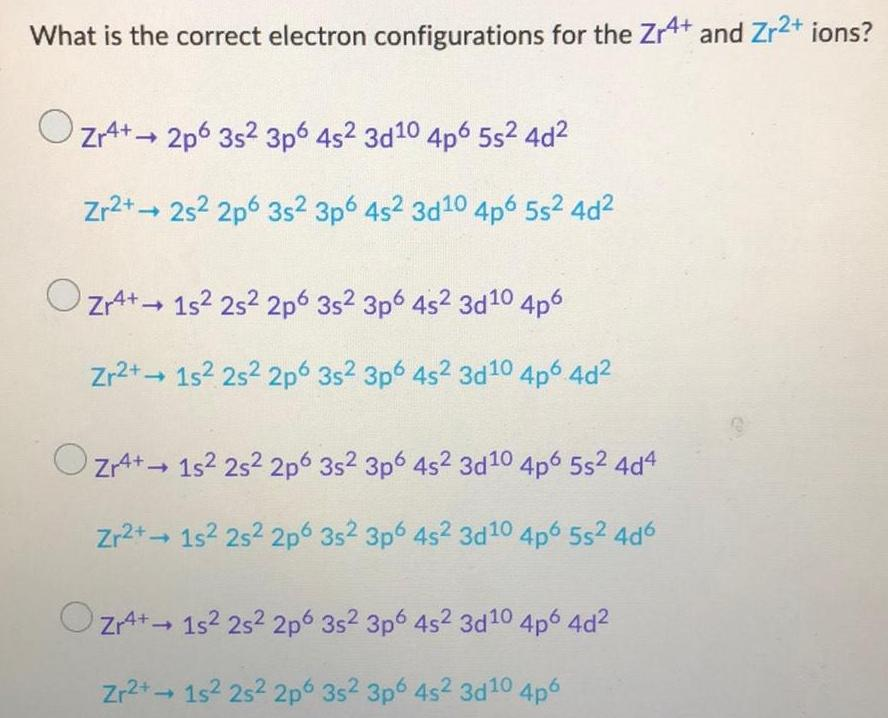Inorganic Chemistry
D Block elements
What is the correct electron configurations for the Zr4+ and Zr²+ ions? Zr4+2p6 35² 3p6 4s² 3d10 4p6 5s² 4d² Zr2+2s² 2p 3s² 3p6 4s2 3d10 4p6 5s² 4d² Zr4+15² 25² 2p 3s² 3p6 4s² 3d10 4p6 Zr2+ 1s² 2s2 2p 3s² 3p6 4s² 3d10 4p6 4d² Zr4+ 15² 25² 2p6 35² 3p6 4s² 3d10 4p6 5s² 4d4 Zr2+1s² 2s² 2p 3s2 3p6 4s2 3d10 4p6 5s²4d6 Zr4+ 1s² 2s² 2p Zr2+→ 1s² 25² 2p 3s² 3p6 4s² 3d¹0 4p6 4d² 3s2 3p6 4s² 3d10 4p6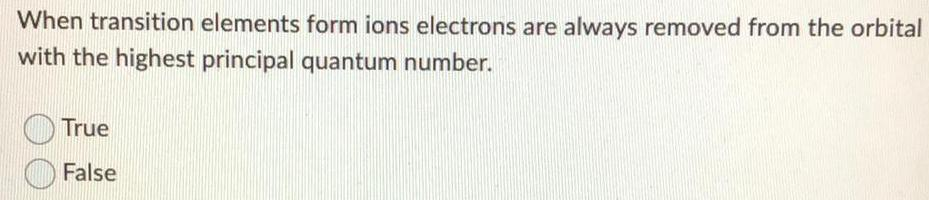Inorganic Chemistry
D Block elements
When transition elements form ions electrons are always removed from the orbital with the highest principal quantum number. True False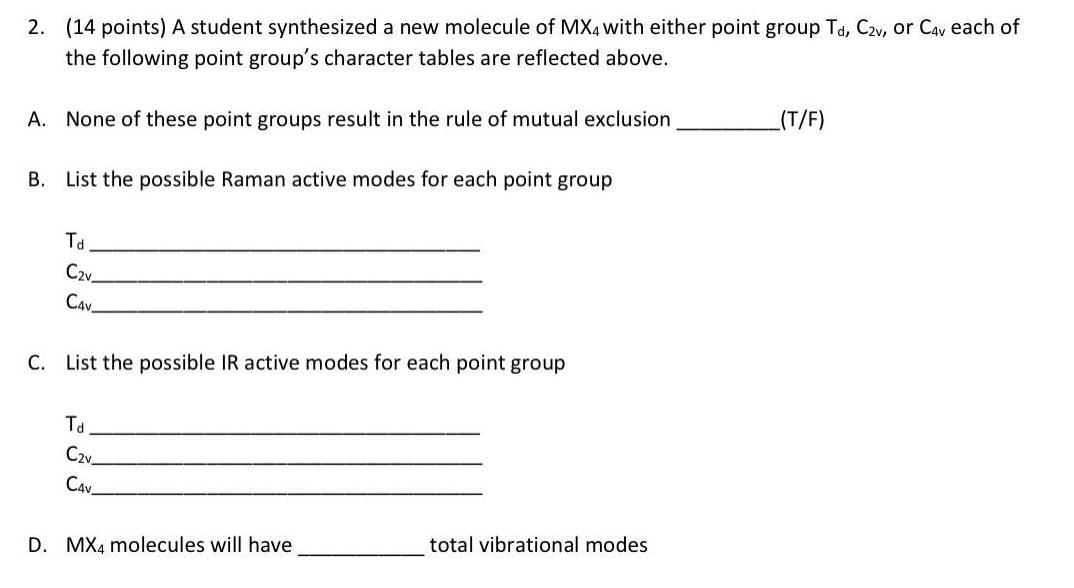Inorganic Chemistry
D Block elements
A student synthesized a new molecule of MX4 with either point group Td, C2v, or C4v each of the following point group's character tables are reflected above. A. None of these point groups result in the rule of mutual exclusion B. List the possible Raman active modes for each point group Td C2v CAV C. List the possible IR active modes for each point group Td C2v C4V D. MX4 molecules will have ____ total vibrational modes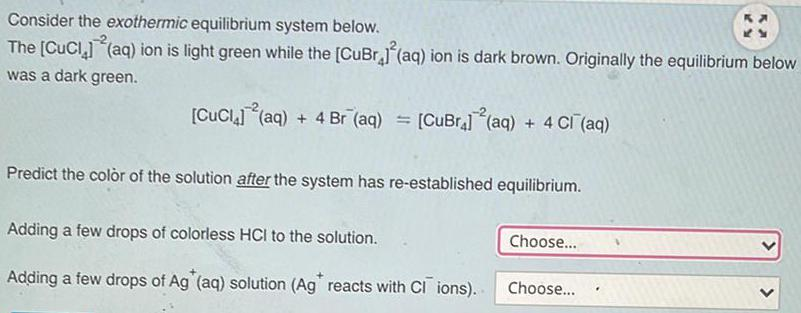Inorganic Chemistry
D Block elements
Consider the exothermic equilibrium system below. The [CuCl4]-2(aq) ion is light green while the [CuBr4]2 (aq) ion is dark brown. Originally the equilibrium below was a dark green. [CuCl4]-2(aq) + 4 Br-(aq) = [CuBr4}-2(aq) + 4 Cl-(aq) Predict the color of the solution after the system has re-established equilibrium. Adding a few drops of colorless HCI to the solution. Adding a few drops of Ag (aq) solution (Ag reacts with CI ions).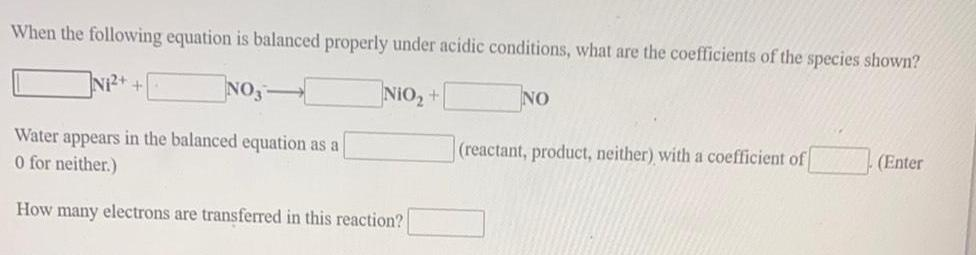Inorganic Chemistry
D Block elements
When the following equation is balanced properly under acidic conditions, what are the coefficients of the species shown? N₁²+ + NO3- NIO₂ + NO Water appears in the balanced equation as a 0 (reactant,product,neither) with a coefficient of (enter 0 or neither) How many electrons are transferred in this reaction?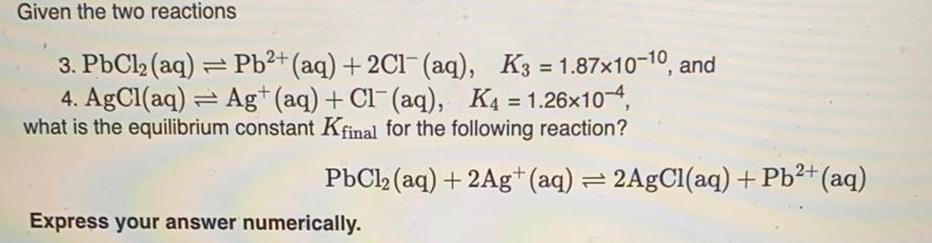Inorganic Chemistry
D Block elements
Given the two reactions 3. PbCl₂ (aq) = Pb²+ (aq) + 2Cl¯ (aq), K3=1.87x10-10, and 4. AgCl(aq) Ag+ (aq) + Cl(aq), K4 = 1.26x10-4, what is the equilibrium constant Kfinal for the following reaction? PbCl₂ (aq) + 2Ag+ (aq) = 2AgCl(aq) + Pb²+ (aq) Express your answer numerically.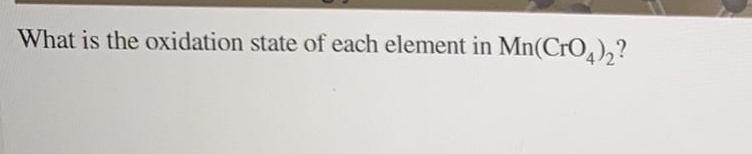Inorganic Chemistry
D Block elements
What is the oxidation state of each element in Mn(CrO4)₂?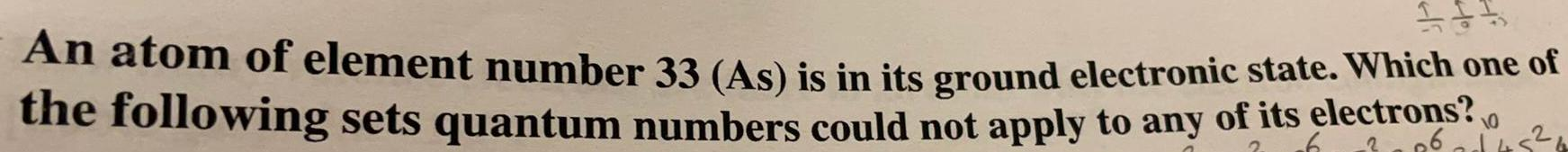Inorganic Chemistry
D Block elements
An atom of element number 33 (As) is in its ground electronic state. Which one of the following sets quantum numbers could not apply to any of its electrons?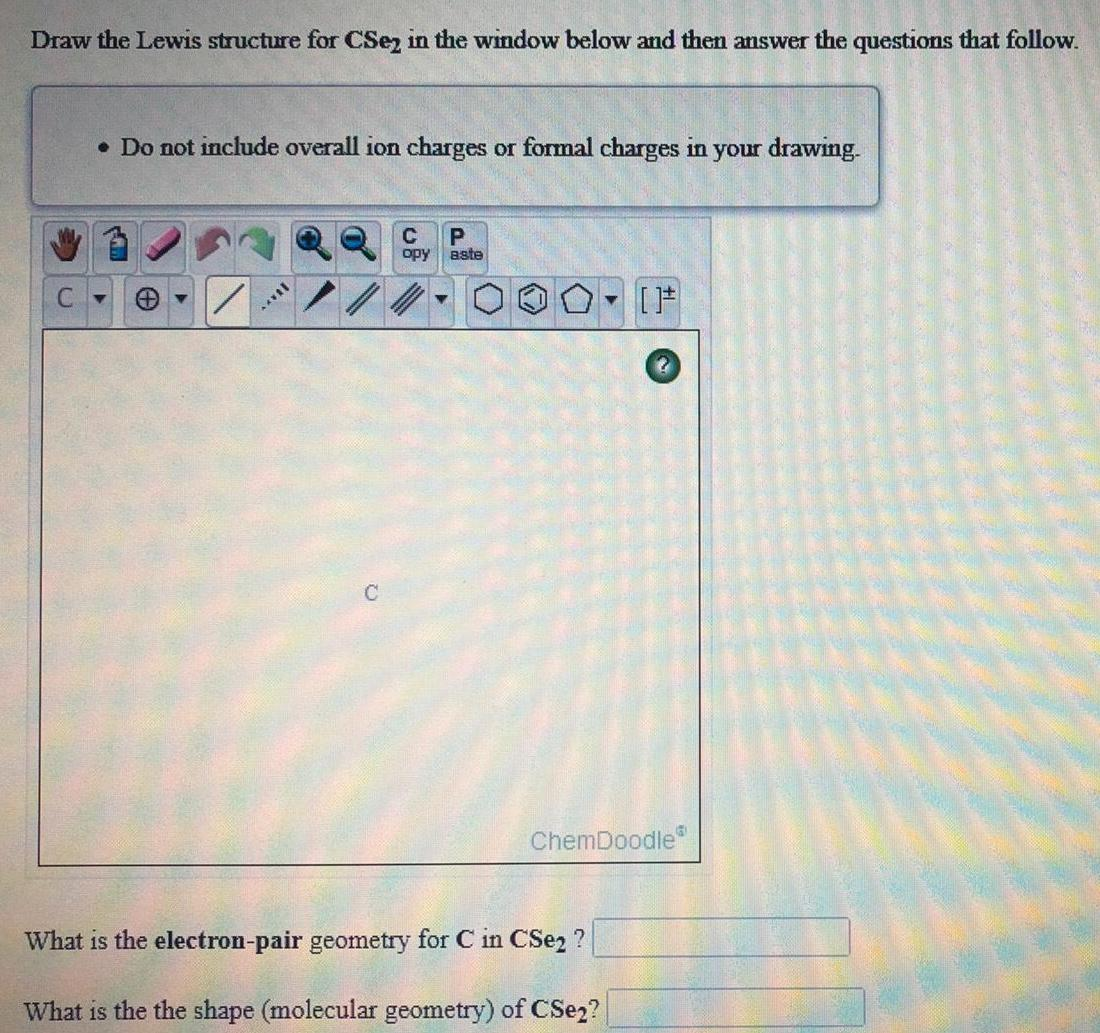Inorganic Chemistry
D Block elements
Draw the Lewis structure for CSe2 in the window below and then answer the questions that follow.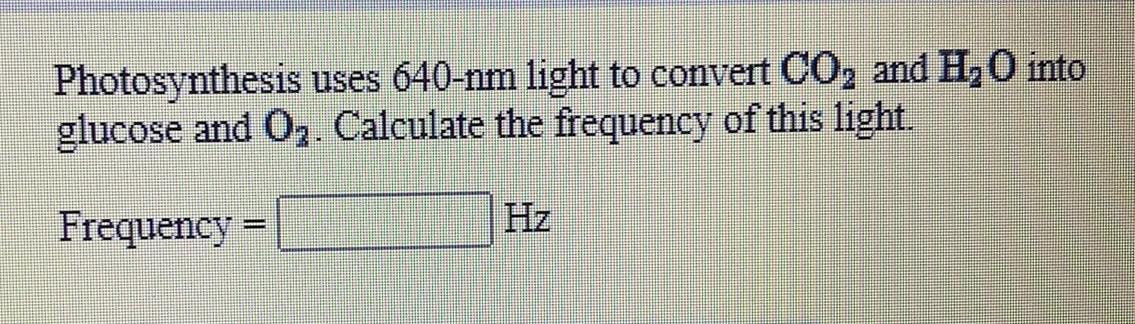Inorganic Chemistry
D Block elements
Photosynthesis uses 640-nm light to convert CO₂ and H₂O into glucose and O₂. Calculate the frequency of this light.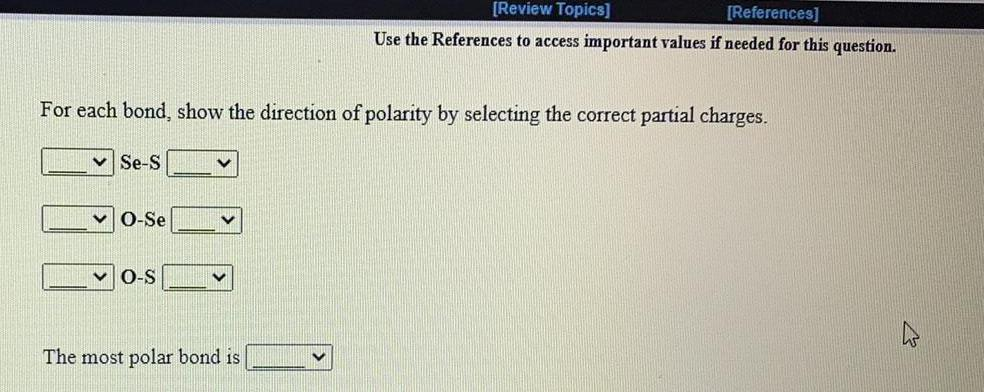Inorganic Chemistry
D Block elements
For each bond, show the direction of polarity by selecting the correct partial charges. Se-S O-Se O-S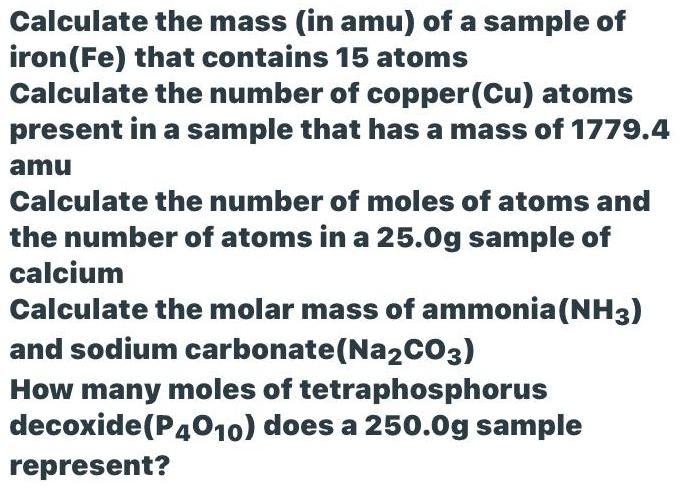Inorganic Chemistry
D Block elements
Calculate the mass (in amu) of a sample of iron (Fe) that contains 15 atoms Calculate the number of copper (Cu) atoms present in a sample that has a mass of 1779.4 amu Calculate the number of moles of atoms and the number of atoms in a 25.0g sample of calcium Calculate the molar mass of ammonia (NH3) and sodium carbonate (Na2CO3) How many moles of tetraphosphorus decoxide (P4010) does a 250.0g sample represent?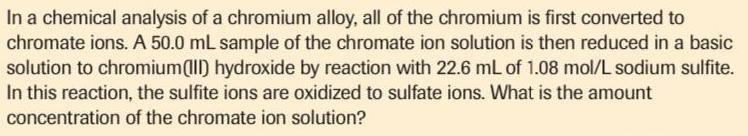Inorganic Chemistry
D Block elements
In a chemical analysis of a chromium alloy, all of the chromium is first converted to chromate ions. A 50.0 mL sample of the chromate ion solution is then reduced in a basic solution to chromium(III) hydroxide by reaction with 22.6 mL of 1.08 mol/L sodium sulfite. In this reaction, the sulfite ions are oxidized to sulfate ions. What is the amount concentration of the chromate ion solution?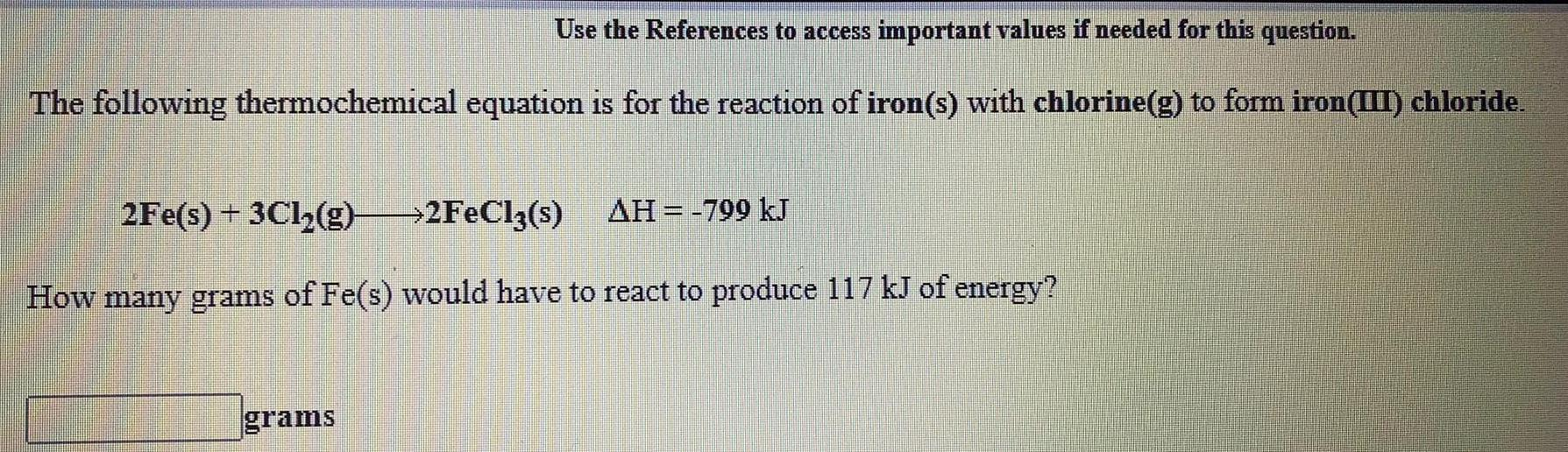Inorganic Chemistry
D Block elements
The following thermochemical equation is for the reaction of iron(s) with chlorine(g) to form iron(III) chloride. 2Fe(s) + 3Cl₂(g) → 2FeCl3(s) ΔH = -799 kJ How many grams of Fe(s) would have to react to produce 117 kJ of energy?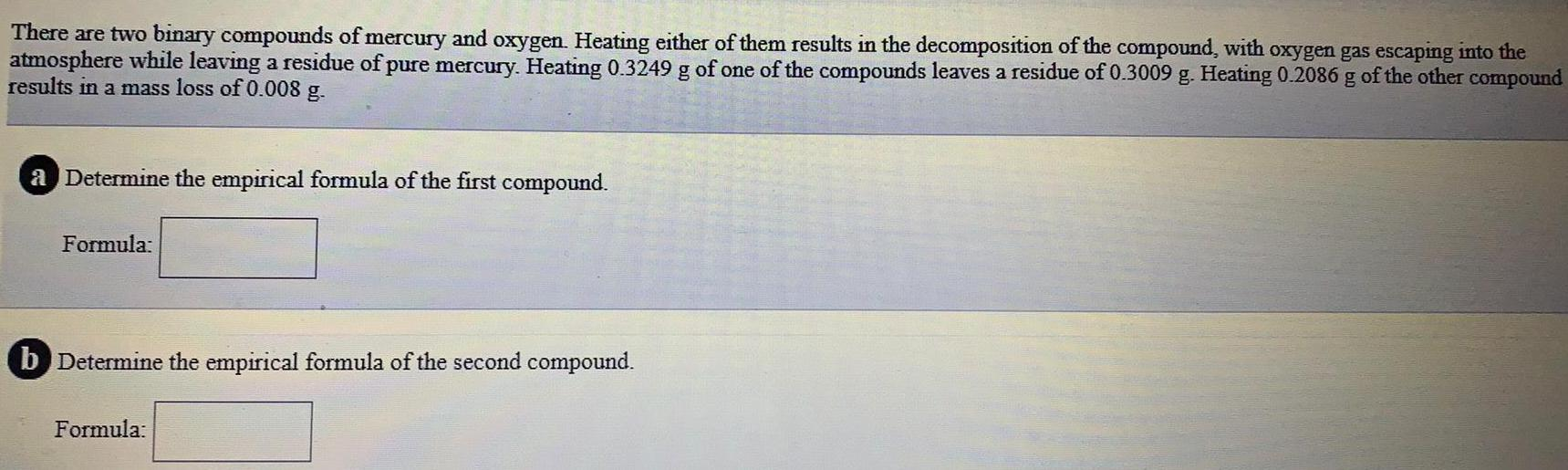Inorganic Chemistry
D Block elements
There are two binary compounds of mercury and oxygen. Heating either of them results in the decomposition of the compound, with oxygen gas escaping into the atmosphere while leaving a residue of pure mercury. Heating 0.3249 g of one of the compounds leaves a residue of 0.3009 g. Heating 0.2086 g of the other compound results in a mass loss of 0.008 g. a Determine the empirical formula of the first compound. Formula: b Determine the empirical formula of the second compound. Formula: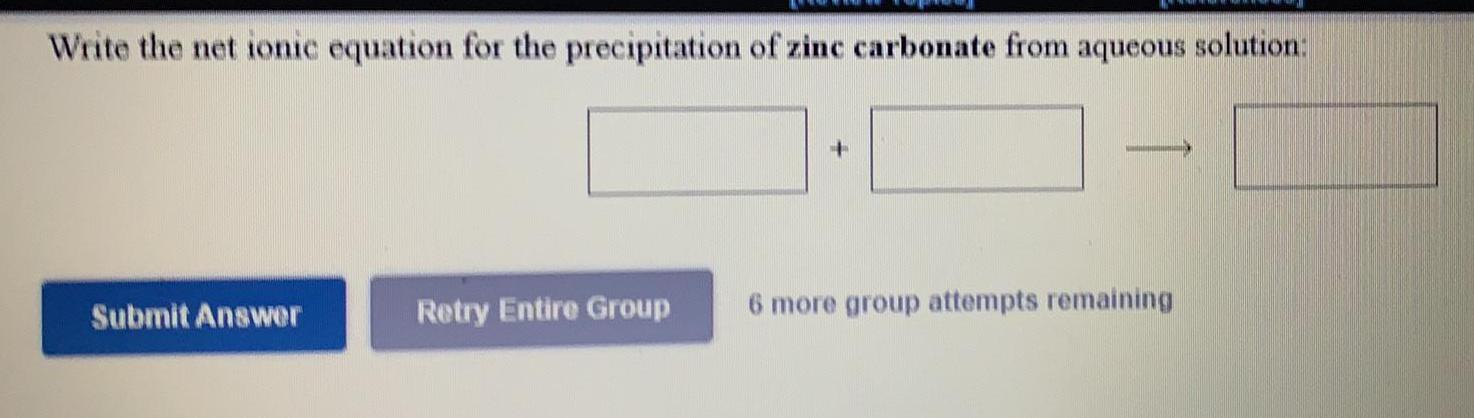Inorganic Chemistry
D Block elements
Write the net ionic equation for the precipitation of zinc carbonate from aqueous solution: